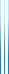# Courses

236602 Theory Lab Winter 2017/2018

This is a discovery-style class, based on work in groups during class time.

The students will work on weekly sheets:

• Week 1 (22 Oct 2017): Fourier analysis: introduction.
• Week 2 (29 Oct 2017): Fourier analysis: influence.
• Week 3 (5 Nov 2017): Fourier analysis: noise.
• Week 4 (12 Nov 2017): Fourier analysis: KKL.
• Week 5 (19 Nov 2017): Information theory: prefix codes.
• Week 6 (26 Nov 2017): Information theory: basic definitions.
• Week 7 (3 Dec 2017): Information theory: applications.
• Week 8 (10 Dec 2017): Information theory: coding.
• Week 9 (24 Dec 2017): Chernoff bound.
• Week 10 (31 Dec 2017): Coding theory: basic notions.
• Week 11 (7 Jan 2018): Coding theory: some codes.
• Week 12 (14 Jan 2018): Linear programming.
• Week 13 (21 Jan 2018): Bonus questions and open discussion.

Bonus sheets:

Possible topics for final assignment (other topics are welcome):

• Fourier analysis:
• Friedgut’s junta theorem and applications or related results.
• $p$-biased analysis and applications, for example the Friedgut–Kalai sharp threshold theorem.
• Gaussian space, the invariance principle, and Majority is Stablest.
• Information theory:
• Pinsker’s inequality and applications.
• Lower bounds in communication complexity.
• Proof of the parallel repetition theorem due to Ankit Garg and Mark Braverman.
• Concentration of measure and large deviation bounds:
• Martingales, Azuma’s inequality, and McDiarmid’s inequality.
• Martingales per se (for example, the optional stopping theorem).
• Coding theory:
• Efficient decoding algorithms.
• List decoding.
• Applications in theory of computation.
• Linear programming:
• Proof of strong duality via Farkas’ lemma.
• Simplex algorithm (and another proof of strong duality).
• Separation oracles.
• Applications to approximation algorithms.
• Semidefinite programming.
• Approximation theory:
• Proof of Markov’s inequality.
• Other applications.
• Algorithms in number theory:
• Primality testing.
• Fast integer multiplication.
• Fast matrix multiplication.
• Integer factorization.
• Polynomial factorization.
• Expander graphs:
• Basic definitions, (double-sided) Cheeger’s inequality, Alon–Boppana.
• Applications, for example Bourgain’s embedding theorem and its tightness.